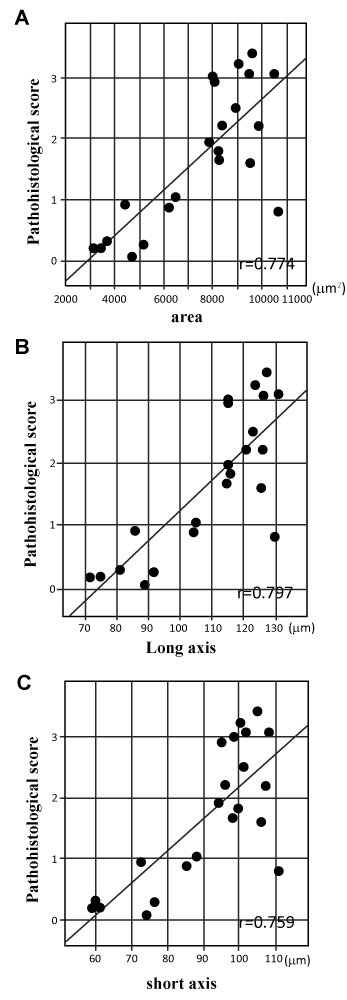Figure 4: Relationship between the mean of the histopathological score of one section and the mean value of the size of glomeruli of same section. (A) There was a positive correlation between the mean of the histopathological score and the mean of area of glomeruli (r = 0.774). (B) Positive correlation between the mean of score and the mean of the long axis (r = 0.797). (C) Positive correlation between the mean of the score and the mean of the long axis (r = 0.759). Regression lines are shown as black lines. p<0.01.